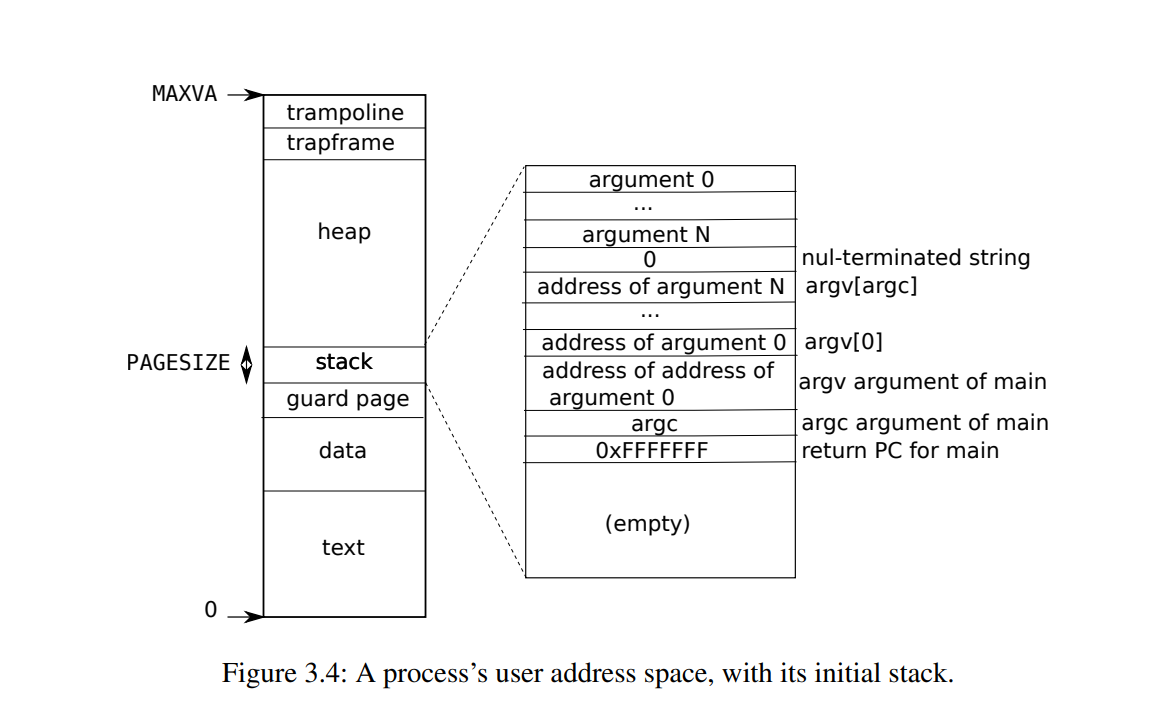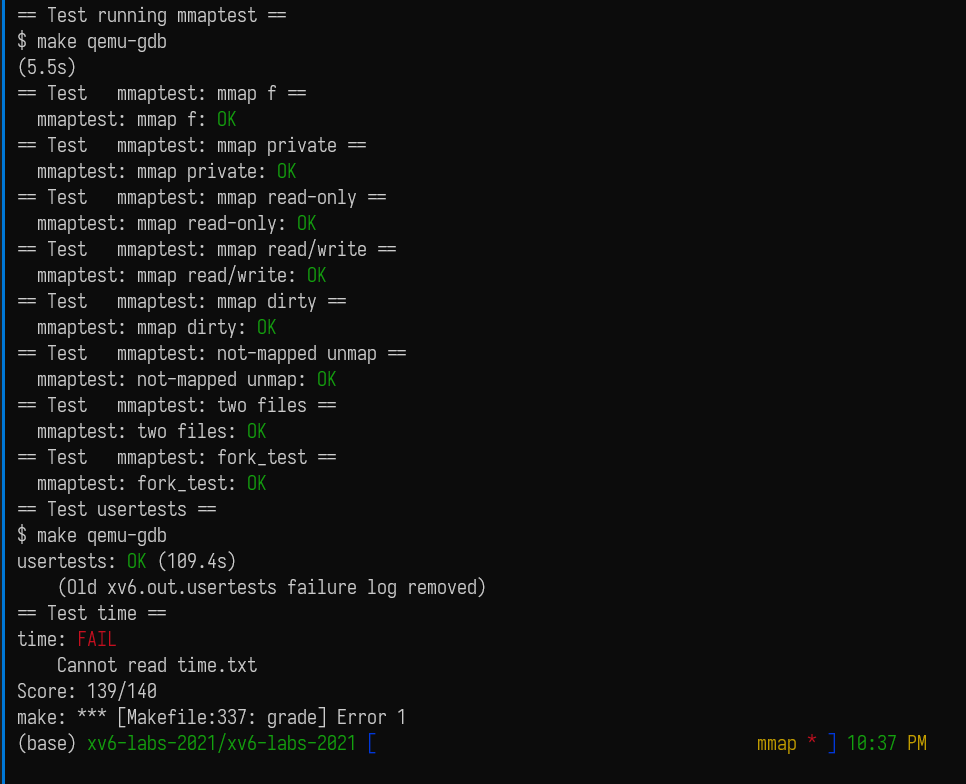upd@2022/9/14：最近把实验的代码放到 github 上了，如果需要参考可以查看这里：

https://github.com/ttzytt/xv6-riscv

# Lab11: mmap

## 描述

mmap() 的定义如下：

void *mmap(void *addr, size_t length, int prot, int flags,
int fd, off_t offset);

protflags 都是一些标志位，具体说，prot 有以下的选项：

• PROT_NONE
• PROT_WRITE
• PROT_EXEC

flags 则决定，如果在内存映射文件中做了修改，是否要在取消映射时，把这些修改更新到文件中。

unmap() 的定义如下：

int munmap(void *addr, size_t length);

## 整体思路uint64
sys_sbrk(void)
{
int n;

if(argint(0, &n) < 0)
return -1;
if(growproc(n) < 0)
return -1;
}

## 代码

struct mmap_vma

// in proc.h
struct mmap_vma{
int in_use;      // 该 vma 结构体是否代表了一个正在使用的文件映射
uint64 sz;       // 映射大小
int prot;
struct file* file; // 映射的文件
int flags;         // map_shared or map_private
};

#define VMA_SZ 16

struct proc {
……
struct mmap_vma mmap_vams[VMA_SZ];
}

sys_mmap()

// in sysfile
uint64
sys_mmap(){
int prot, flags, fd;
struct file* file;
//void *mmap(void *addr, size_t length, int prot, int flags, int fd, off_t offset);
// 这参数是真的多。。
try(argint(2, &prot), return -1)
try(argint(3, &flags), return -1)
try(argfd(4, &fd, &file), return -1) // 同时取得文件和描述符
// 读入参数
struct proc* p = myproc();
if(addr || offset) // 我们实现的是 mmap 的子集，不支持自定内存和偏移量
return -1;
if(!file->writable && (prot & PROT_WRITE) && (flags & MAP_SHARED))
return -1;
// 如果文件本身不允许写入，但 PROT_WRITE 还是设置了

int unuse_idx = -1;
uint64 sta_addr = get_mmap_space(length, p->mmap_vams, &unuse_idx);

if(unuse_idx == -1)
return -1;
if(sta_addr <= p->sz) // 没内存来 mmap 了
return -1;
struct mmap_vma* cur_vma = &p->mmap_vams[unuse_idx];
cur_vma->file = file;
cur_vma->in_use = 1;
cur_vma->prot = prot;
cur_vma->flags = flags;
cur_vma->sz = length;
filedup(file); // 增加引用计数
} 

get_mmap_space()// in sysfile.c
uint64
get_mmap_space(uint64 sz, struct mmap_vma* vmas, int* free_idx){
*free_idx = -1;

// 返回一个可以储存新文件映射的地址（开始地址）
// 优先查看 vma 槽中的“空隙”，如果没有，那就映射到最下面
// 其实可以写一个快速排序，但是我懒。。。

struct mmap_vma tmp; // 作为上边界，可能和上图一样，最上方没有任何映射区域
tmp.sta_addr = TRAPFRAME, tmp.sz = 0;

for(int i = 0; i <= VMA_SZ; i++){
// 假设 vmas[i] 的 PGROUNDDOWN(sta_addr) 是新文件映射的结束位置
if(vmas[i].in_use == 0 && i != VMA_SZ){
*free_idx = i;
continue;
}
uint64 ed_pos = i != VMA_SZ ? PGROUNDDOWN(vmas[i].sta_addr)

for(int j = 0; j < VMA_SZ; j++){
// 假设 vmas[j] 的 sta_addr + sz（vma[j] 的结束位置） 往上是新映射的起始位置
if(vmas[j].in_use == 0 && i != VMA_SZ) continue;

uint64 st_pos = i != VMA_SZ ? vmas[j].sta_addr + vmas[j].sz
: tmp.sta_addr + tmp.sz; // 这个位置一定是页对齐的

if (ed_pos <= st_pos) continue;
// 这里直接跳过，不在下面判断是因为无符号类，如果做下面的减法会出错
if (ed_pos - st_pos >= sz){
// [st_pos, ed_pos) 的区间
return st_pos;
}
}
}

}

mmap_fault_handler()

// in trap.c
int
struct proc* p = myproc();
struct mmap_vma* cur_vma;
// 找到这个地址属于哪个文件的映射
// 等于零说明不属于任何一个
return -1;
}

if(!cur_vma->file->readable && r_scause() == 13 && cur_vma->flags & MAP_SHARED){
return -1;
} // 读错误

if(!cur_vma->file->writable && r_scause() == 15 && cur_vma->flags & MAP_SHARED){
DEBUG("mmap_fault_handler: not writable\n");
return -1;
} // 写错误

uint64 pa = kalloc();
if(!pa){
DEBUG("mmap_fault_handler: kalloc failed\n");
return -1;
}

memset(pa, 0, PGSIZE);

int perm = PTE_U | PTE_V;
if(cur_vma->prot & PROT_READ) perm |= PTE_R;
if(cur_vma->prot & PROT_WRITE) perm |= PTE_W;
if(cur_vma->prot& PROT_EXEC) perm |= PTE_X;
// 在 mmap 的时候已经排除了不可能的情况了

// 这个 off 代表文件拷贝时要跳过多少个页帧

ilock(cur_vma->file->ip);
int rdret;
if((rdret = readi(cur_vma->file->ip, 0, pa, off, PGSIZE)) == 0){
iunlock(cur_vma->file->ip);
return -1;
}

iunlock(cur_vma->file->ip); // 没有 put 是这个文件之后还需要使用
// 在 unmap 中应该可以 put
mappages(p->pagetable, pg_sta, PGSIZE, pa, perm);
return 0;
}

get_vma_by_addr()

struct mmap_vam*
struct proc* p = myproc();
for(int i = 0; i < VMA_SZ; i++){
// 判断该地址是否在文件映射区的中间
return p->mmap_vams + i;
}
}
return 0;
}

usertrap()

// in trap.c
……
if(r_scause() == 8){
// system call

if(p->killed)
exit(-1);

// sepc points to the ecall instruction,
p->trapframe->epc += 4;

// an interrupt will change sstatus &c registers,
// so don't enable until done with those registers.
intr_on();

syscall();
} else if((which_dev = devintr()) != 0){
// ok
} else if ((r_scause() == 13 || r_scause() == 15)){
}
else{
}

printf("usertrap(): unexpected scause %p pid=%d\n", r_scause(), p->pid);
printf("            sepc=%p stval=%p\n", r_sepc(), r_stval());
p->killed = 1;
}
……

#define PTE_D (1L << 7)

• unmap 的部分可能没有跨过一个页帧，也就是说 unmap 的所有内存都在一个页帧之内，那么这个页帧不能释放，但是需要把内存中的数据复制回文件。
• 对于 unmap 结尾地址在某个页帧中间的情况，需要分类讨论。如果结尾是中间，但这个页帧是映射区的最后一个页帧，那既需要释放页帧，也许要写回文件。如果是中间，但不是最后一个页帧，那就不能释放。

// in vm.c
int
mmap_writeback(pagetable_t pt, uint64 src_va, uint64 len, struct mmap_vma* vma){
// 把带脏位的页帧写回文件中，并且取消映射
// 写回的是 src_va 开始的，长度为 len
uint64 a;
pte_t *pte;
for(a = PGROUNDDOWN(src_va); a < PGROUNDDOWN(src_va + len); a += PGSIZE){
if((pte = walk(pt, a, 0)) == 0){
panic("mmap_writeback: walk");
}
if(PTE_FLAGS(*pte) == PTE_V)
panic("mmap_writeback: not leaf");
if(!(*pte & PTE_V)) continue; // 懒分配

if((*pte & PTE_D) && (vma->flags & MAP_SHARED)){
// 写回
begin_op();
ilock(vma->file->ip);
uint64 copied_len = a - src_va;
writei(vma->file->ip, 1, a, copied_len, PGSIZE);
iunlock(vma->file->ip);
end_op();
}
kfree(PTE2PA(*pte));
*pte = 0;
}
return 0;
}

//in vm.c
int
mmap_writeback_na(pagetable_t pt, uint64 src_va, uint64 len, struct mmap_vma* vma){
uint64 a;
pte_t *pte;
a = PGROUNDDOWN(src_va);

if(a == PGROUNDDOWN(src_va + len)){
// 如果 unmap 的部分在一个页帧的范围内
begin_op();
ilock(vma->file->ip);
writei(vma->file->ip, 1, src_va, 0, src_va - a);
iunlock(vma->file->ip);
end_op();
}

for(; a < PGROUNDDOWN(src_va + len); a += PGSIZE){ // 这部分只处理整页
// 如果结尾停在页中间不会处理
if((pte = walk(pt, a, 0)) == 0){
panic("mmap_writeback: walk");
}
if(PTE_FLAGS(*pte) == PTE_V)
panic("mmap_writeback: not leaf");
if(!(*pte & PTE_V)) continue; // 懒分配
if((*pte & PTE_D) && (vma->flags & MAP_SHARED)){
// 写回
begin_op();
ilock(vma->file->ip);
// 第一次的时候，a 会比 src_va 小
uint64 copied_len = a - src_va;
if(a < src_va){
// 第一个页帧，不是完整的
// 这种情况也需要 kfree，因为跨过了一个页帧
writei(vma->file->ip, 1, src_va, 0, src_va - a);
} else {
writei(vma->file->ip, 1, a, copied_len, PGSIZE);
}
iunlock(vma->file->ip);
end_op();
}
kfree(PTE2PA(*pte));
*pte = 0;
}

uint64 copied_len = a - src_va;
uint64 len_left = vma->sz - copied_len;

if (len_left){
// 处理 unmap 结尾在页帧中间的情况
begin_op();
ilock(vma->file->ip);
writei(vma->file, 1, a, copied_len, len_left);
if(len_left + a == vma->sz + src_va){ // 如果停在的页帧刚好是最后一个
pte_t *pte;
if((pte = walk(pt, a, 0)) == 0){
panic("mmap_writeback: walk");
}
kfree(PTE2PA(*pte));
}
iunlock(vma->file->ip);
end_op();
}

return 0;
}

// in sysfile.c
uint64
struct proc* p = myproc();
if(!cur_vma)
return -1;

// 从中间挖洞
return -1;
}

// 从起始位置删除的
}
cur_vma->sz -= len;

if(cur_vma->sz <= 0){
// 如果整个映射区都没了
fileclose(cur_vma->file);
cur_vma->in_use = 0;
}
return 0;
}

uint64
sys_munmap(){
// int munmap(void *addr, size_t length);
uint64 len;
}

// in proc.c wait():
……
for(;;){
// Scan through table looking for exited children.
havekids = 0;
for(np = proc; np < &proc[NPROC]; np++){
if(np->parent == p){
// make sure the child isn't still in exit() or swtch().
acquire(&np->lock);

havekids = 1;
if(np->state == ZOMBIE){
// Found one.
pid = np->pid;
sizeof(np->xstate)) < 0) {
release(&np->lock);
release(&wait_lock);
return -1;
}
freeproc(np); // 注意这里，只有父进程 wait 的时候才会去 freeproc。
release(&np->lock);
release(&wait_lock);
return pid;
}
release(&np->lock);
}
}
……
}
……

// in proc.c exit():
void
exit(int status)
{
struct proc *p = myproc();

if(p == initproc)
panic("init exiting");

// 释放和写回 mmap 数据需要在关闭文件之前
for(int i = 0; i < VMA_SZ; i++){
if(p->mmap_vams[i].in_use){
}
}

// Close all open files.
for(int fd = 0; fd < NOFILE; fd++){
if(p->ofile[fd]){
struct file *f = p->ofile[fd];
fileclose(f);
p->ofile[fd] = 0;
}
}
……
}

fork()

// in proc.c
……
for (int i = 0; i < VMA_SZ; i++){
if(p->mmap_vams[i].in_use){
np->mmap_vams[i] = p->mmap_vams[i];
filedup(p->mmap_vams[i].file);
// 复制 vma
}
}
……

// in vm.c
for(i = 0; i < sz; i += PGSIZE){ // 注意这里范围
if((pte = walk(old, i, 0)) == 0)
panic("uvmcopy: pte should exist");
if((*pte & PTE_V) == 0)
panic("uvmcopy: page not present");
pa = PTE2PA(*pte);
flags = PTE_FLAGS(*pte);
if((mem = kalloc()) == 0)
goto err;
memmove(mem, (char*)pa, PGSIZE);
if(mappages(new, i, PGSIZE, (uint64)mem, flags) != 0){
kfree(mem);
goto err;
}
}## 吐槽

CFLAGS = -Wall -O -g3 -fno-omit-frame-pointer -ggdb -UFDEBUG

\$ usertests writebig
usertests starting
test writebig: panic: balloc: out of blocks

https://github.com/mit-pdos/xv6-riscv/issues/59